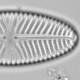## Independent adventures in uncertainty: sea-ice reconstructions

One of the assumptions of transfer functions is that the calibration set observations are independent. If they are not independent of each other, for example because of spatial autocorrelation, the transfer function performance statistics will be biased, appearing to be better than is justified by the data (see my autocorrelation papers for details).

The modern analogue technique (MAT) is particularly prone to biased performance statistics if there is spatial autocorrelation. During cross-validation, sites that are geographically close to the test observation are often selected as analogues. Ideally, these neighbouring analogues are selected because they are similar values of the environmental variable being reconstructed. However, if the environmental variable was ecologically irrelevant, neighbouring sites might be selected as analogues because they are similar in other environmental variables. In this case, the cross-validated predictions would still be good, but the model would have no predictive power. Most real transfer functions in spatially autocorrelated environments lie somewhere between these two extremes.

If a transfer function can make good predictions on a spatially independent test set, then one can be confident that it has real predictive power. The test is to divide the calibration set into regions and for each region calculate the uncertainty of cross-validation predictions and the uncertainty of predictions from a model trained on the other regions. If autocorrelation is a minor issue, then the cross-validation performance and the performance of the spatially independent model should be similar. This is a powerful test of a bias in transfer function performance caused by spatial autocorrelation.

The circum-Arctic 1171-observation dinocyst calibration set can be divided into three sectors: one region centred on the Nordic Seas, another on Baffin Bay and Hudson Bay, and the third on the Bering Strait. Each region spans the same range of sea ice coverage. I’ve only used the sites with a winter SST below 7°C, to reduce the bias caused by the uneven distribution of sites along the gradient. It is very unlikely that the new 1492-observation calibration would yield substantially different results from those below.Red lines separate the dinocyst observations into three regions. Only the sites with winter SST below 7°C (blue) are considered.

I’ve fitted MAT using the approach usually used by dinocyst workers, but have not omitted poor analogues.

For each region, the cross-validation predictions have a much smaller error than predictions from models trained on the other two regions.

Error in predictions under cross-validation and as an independent test set
Region Cross-validation RMSEP RMSEP as independent test set
Nordic Seas 1.47 2.91
Baffin Bay 0.84 2.84
Bering Strait 0.84 2.80

This is not encouraging. Note, however, that the independent RMSEP is still lower than the standard deviation (about 4 months) in each region – the models do have some skill. This skill could arise because the dinoflagellates genuinely care about sea ice, or because they really care about, for example, summer temperature and this is correlated with sea-ice coverage.

The increase in error could be because of endemic species in each region, but I don’t think endemic species are likely to be important in the circum-Arctic area. It could be because some other environmental variable is very different between the different regions. Or it could be that the cross-validation estimates are heavily biased by spatial autocorrelation. Whatever the cause, the general relationship between dinocyst assemblages and sea ice is not strong.

If the models cannot make good predictions in space, they cannot be trusted to make good prediction in time: “The past is a foreign country, they do things differently there”.

The increase in error shown probably includes most of the increase in error due to the uneven sampling of the gradient. These two sources of bias will not be independent.

The precision of the dinocyst sea-ice reconstructions needs to be questioned. If the error is 2-3 times large than reported by de Vernal et al (2013), their utility is probably minimal.

R-code below the fold.

```library(rioja)
library(maps)
library(mapproj)
dat\$X<-NULL
names(dat)
meta<-dat[,c(1:3, 80:91)]
spp<-dat[,-c(1:3, 80:91)]
spp<-spp/rowSums(spp)
sppt<-log(spp*1000+1)

#map
x11();par(mar=rep(0.1,4))
map(ylim=c(10,90), proj="azequalarea")
points(mapproject(meta\$LONG, meta\$LAT), col=ifelse(meta\$Winter.SST<7, "blue", "pink"), pch=20)
lines(mapproject(x=c(0,-43), y=c(90,45),), col=2, lwd=2)
lines(mapproject(x=c(0,95), y=c(90,70),), col=2, lwd=2)
lines(mapproject(x=c(0,-120), y=c(90,45),), col=2, lwd=2)

#define regions
nordic<-meta\$LONG>=-43&meta\$LONG<95&meta\$Winter.SST<7
baffin<-meta\$LONG>=-120&meta\$LONG<(-43)&meta\$Winter.SST<7
bering<-(meta\$LONG<(-120)|meta\$LONG>=95)&meta\$Winter.SST<7

x11();par(mfrow=c(3,1), mar=c(3,3,1,1), mgp=c(1.5,.5,0))
hist(meta\$Sea.ice[nordic])
hist(meta\$Sea.ice[baffin])
hist(meta\$Sea.ice[bering])

#everything
mod0<-MAT(sppt[meta\$Winter.SST<7,], meta\$Sea.ice[meta\$Winter.SST<7], dist="euclidean")
performance(mod0)

quantile(paldist(sppt[meta\$Winter.SST<7,], dist="euclidean"), prob=c(0.05, 0.1))

#nordic only
mod.nordic<-MAT(sppt[nordic,], meta\$Sea.ice[nordic], dist="euclidean")
performance(mod.nordic)
#baffin only
mod.baffin<-MAT(sppt[baffin,], meta\$Sea.ice[baffin], dist="euclidean")
performance(mod.baffin)
#bering only
mod.bering<-MAT(sppt[bering,], meta\$Sea.ice[bering], dist="euclidean")
performance(mod.bering)

#Not nordic
mod.Nnordic<-MAT(sppt[baffin|bering,], meta\$Sea.ice[baffin|bering], dist="euclidean")
pred.nordic<-predict(mod.Nnordic, sppt[nordic,])
summary(pred.nordic\$dist.n)
sqrt(mean((meta\$Sea.ice[nordic]-pred.nordic\$fit[,2])^2))

#not baffin
mod.Nbaffin<-MAT(sppt[bering|nordic,], meta\$Sea.ice[bering|nordic], dist="euclidean")
pred.baffin<-predict(mod.Nbaffin, sppt[baffin,])
summary(pred.baffin\$dist.n)
sqrt(mean((meta\$Sea.ice[baffin]-pred.baffin\$fit[,2])^2))

#not bering
mod.Nbering<-MAT(sppt[baffin|nordic,], meta\$Sea.ice[baffin|nordic], dist="euclidean")
pred.bering<-predict(mod.Nbering, sppt[bering,])
summary(pred.bering\$dist.n)
sqrt(mean((meta\$Sea.ice[bering]-pred.bering\$fit[,2])^2))

sd(meta\$Sea.ice[bering])
```